KCET 2016 solved Physics paper will help the students to prepare efficiently for the KCET Physics exam by understanding the allocation of marks for different topics across the subject. Practising KCET previous year question papers with answer keys will help the aspirants to face the exam confidently. This set of solutions is designed to help the candidates in achieving time management skills and also acts as an effective aid in their preparation.

### KCET 2016 - Physics

Question 1: A body falls freely for 10 sec. Its average velocity during this journey (take g = 10 ms-2)

1. a. 100 ms-1
2. b. 10 ms-1
3. c. 50 ms-1
4. d. 5 ms-1

Solution:

Given:-

g = 10 m/s2

t = 10 sec

Initial velocity (u) = 0 m/s

S = ut + (1 / 2) gt2

S = 0 + (1 / 2) ×10 × 102

Vavg. = 500 / 10 = 50 m/s

Question 2: Three projectiles A, B and C are projected at an angle of 30°, 45°, 60° respectively. If RA, RB, and RC are ranges of A, B and C respectively, then (velocity of projection is the same for A, B & C).

1. a. RA = RB = RC
2. b. RA = RC > RB
3. c. RA < RB < RC
4. d. RA = RC < RB

Solution:

RA = RC < RB

R = u2 sin θ / g

RA = u2 sin 60o / g = (√3 / 2) (u2 / g), RB = u2 sin 90o / g = (u2 / g)

RC = u2 sin 120o / g = (u2 / g) cos 30o = (u2 / g) × (√3 / 2)

Therefore, RA = RC < RB

Question 3: The component of a vector r along x-axis has a maximum value if

1. a. r is along + ve x-axis
2. b. r is along with + ve y-axis
3. c. r is along – ve y-axis
4. d. r makes an angle of 45° with the x-axis

Solution: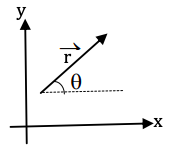rx = |r|cos θ

(rx) = |r| cos max

rx = |r| cos θ

cos θ is maximum of θ = 0

θ = 0

r is along +ve x-axis

Question 4: Maximum acceleration of the train in which a 50 kg box lying on its floor will remain stationary (Given: Coefficient of static friction between the box and the floor of the train is 0.3 and g = 10 ms-2)

1. a. 5.0 ms-2
2. b. 3.0 ms-2
3. c. 1.5 ms-2
4. d. 15.0 ms-2

Solution:

μ = 0.3

m = 50 kg

g = 10 m/s2

f = μmg

The box kept on the floor of the train remains stationary if the pseudo force acting on the box is balanced by frictional force.

ma = μmg

a = μg = 0.3 × 10 = 3.0 m/s2

Question 5: A 12 kg bomb at rest explodes into two pieces of 4 kg and 8 kg piece is 20 Na, the kinetic energy of the 8 kg piece is -

1. a. 25 J
2. b. 20 J
3. c. 50 J
4. d. 40 J

Solution:

The initial momentum of the system is zero.

According to the law of conservation of momentum.

Initial momentum = final momentum

So, the final momentum of the system must also be zero.

Hence, the momentum of an 8kg piece must be equal, opposite to the momentum of a 4kg piece.

8kg. piece, P = 20 NS

K.E. of 8kg piece, K = P2 / 2m

(20)2 / (2 * 8) = 25 J

Question 6: Which of the points is likely the position of the centre of mass of the system shown in the figure?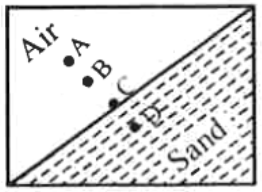1. a. A
2. b. D
3. c. B
4. d. C

Solution:

The centre of mass lies towards the heavier mass, from the above diagram, the mass is more at point D.

Question 7: Three bodies, a ring (R), a solid cylinder (C) and a solid sphere (S) having the same mass and same radius roll down the inclined plane without slipping. They start from rest if vR, vC, and vS are velocities of respective bodies on reaching the bottom of the plane, then -

1. a. vR = vC = vS
2. b. vR > vC > vS
3. c. vR, vC < vS
4. d. vR = vC > vS

Solution:

Let the body start from rest

Using the work-energy equation

W = Δ K.E

= K.Ef – K.Ei

= K.E.f [K.Ei = 0]

mgh = (1 / 2) mv2 + (1 / 2) Iω2

But V = r ω

I = kmr2

mgh = (1 / 2) mv2 + (1 / 2) kmv2

mgh = (1 / 2) mv2 [1 + k]

v = √2gh / (1 + k)

As we know; kR = 1, kC = 0.5, kS = 0.4

KS < KC < KR

VR < VC < VS

Question 8: Variation of acceleration due to gravity (g) with distance x from the centre of the earth is best represented by (R → Radius of the earth)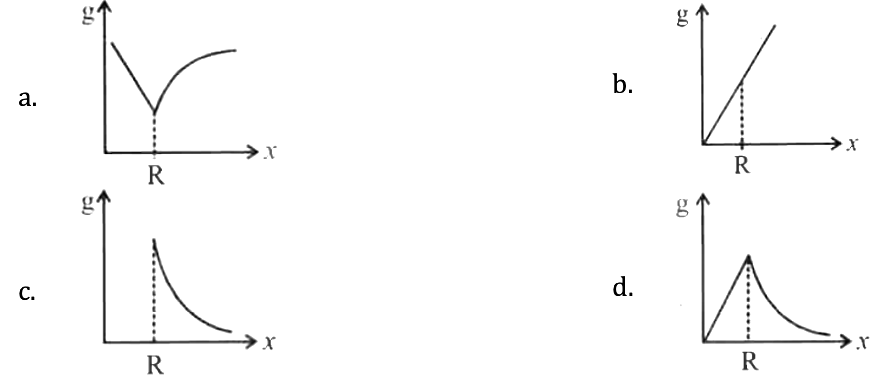Solution:

Acceleration due to gravity at a depth d

g = g0(1 - [d / R])

Distance from earth centre x = R - d

d = R - x

g = g0 (x / R)

Thus acceleration due to gravity increases linearly with the increase in distance from the centre of the earth.

Acceleration due to gravity at a height h

g = g0 (R / [R + h])2

Distance from earth centre x = R + h

h = x – R

g = g0 (R / x)2

So acceleration due to gravity decreases as (1 / x2) with the increase in distance from the centre of earth.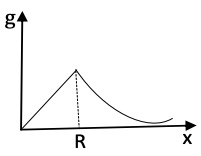Question 9: A spring is stretched by applying a load to its free end. The strain produced in the spring is –

1. a. Volumetric
2. b. Shear
3. c. Longitudinal & Shear
4. d. Longitudinal

Solution:

The length and shape of the spring changes and the weight of the load behave as a deforming force. The change in length corresponds to longitudinal strain and change in shape corresponds to shearing strain.

Question 10: An ideal fluid flow through a pipe of the circular cross-section with diameters 5 cm and 10 cm as shown. The ratio of velocities of fluid at A and B is –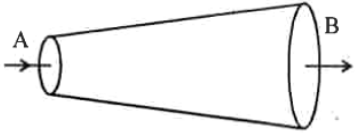1. a. 4 : 1
2. b. 1 : 4
3. c. 2 : 1
4. d. 1 : 2

Solution: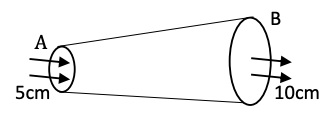DA = 5cm, DB = 10cm

From continuity equation

A1V1 = A2V2

Where,

V1 = VA

V2 = VB

[πD2A / 4] VA = [πD2B / 4] VB

VA / VB = D2B / D2A = 102 / 52

On solving

VA / VB = 4 / 1

Question 11: A pan filled with hot food from 94°C to 86°C in 2 minutes. When the room temperature is 20°C. How long will it cool from 74°C to 66°C?

1. a. 2 minutes
2. b. 2.8 minutes
3. c. 2.5 minutes
4. d. 1.8 minutes

Solution:

Using newton’s law of cooling with approximation

dT / dt = - k / ms (Tavg – Tsurrounding) … (1)

Case I:- dt = 2min, Ts = 20°C

Tavg = [94 + 86] / 2 = 90°C

dT = 94 – 86 = 8°C

- k / ms = [dT / dt] × [1 / (Tavg - Ts)]

= (8 / 2) × (1 / [90 - 70])

= 0.05714

Case II:-

Ts = 20°C, Tavg = [74 + 66] / 2 = 70oC

dT = 74 - 66 = 8°C

Now value’s putting in equation (1)

(8 / dt) = 0.05714 × (70 – 20)

dt = 2.8 min

Question 12: Four rods with different radii r and length l are used to connect to heat reservoirs at different temperatures. Which one will conduct most heat?

1. a. r = 1 cm, l = 2 m
2. b. r = 1 cm, l = (1 / 2) m
3. c. r = 2 cm, l = 2 m
4. d. r = 2 cm, l = (1 / 2) m

Solution:

Heat conducted by Rod

Q = [KAt ΔT] / l

A = πr2

So,

The rate of the flow of heat

dθ / dt ∝ [AΔT / l]

On considering the above relation

r = 2 cm, l = (1 / 2) m

Question 13: A Carnot engine working between 300 K and 400 K has 800 J of useful work. The amount of heat energy supplied to the engine from the source is –

1. a. 2400 J
2. b. 3200 J
3. c. 1200 J
4. d. 3600 J

Solution:

Given:-

TM = 400k

TL = 300k

W = 800J

We know

Efficiency (𝜂) = 1 - (TL / TH) = W / Q

1 - (300 / 400) = 800 / Q

On solving Q = 3200 J

Question 14: A particle executing SHM has a maximum speed of 0.5 ms-1 and a maximum acceleration of 1.0 ms-2. The angular frequency of oscillation is –

Solution:

Maximum velocity, Vmax = ωA = 0.5 m/s

Maximum acceleration, amax = ω2A = 1 m/s2

Angular frequency ω = amax / vmax

ω = 1 / 0.5

Question 15: A source of sound is moving with a velocity of 50 ms-1 towards the stationary observer. The observer measures the frequency of sound as 500 Hz. The apparent frequency of sound as heard by the observer when the source is moving away from him with the same speed is (Speed of sound at room temperature 350 ms-1) –

1. a. 400 Hz
2. b. 666 Hz
3. c. 375 Hz
4. d. 177.5 Hz

Solution:

Speed of source, Vs = 50m/s

V = 350m/s [speed of sound]

Using Doppler formula:-

f ‘ = {[v + v0] / [v - vs]} f ---- (1)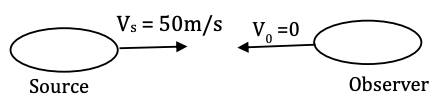Given:- Frequency Heard by the observer (f’’) = 500Hz

From equation (1)

500 = {[350 + 0] / [350 - 50]} f

500 = (35 / 30) f

f = 15000 / 35

f = 428.6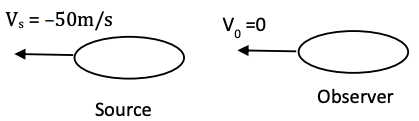Frequency heard observer is (f’’) = {[350 + 0] / [350 + 50]} 428.6

= (35 / 40) 428.6

= 375Hz

Question 16: If there is only one type of charge in the universe, then (E → Electric field, ds → Area vector)

1. a. ∮E . ds ≠ 0 on any surface
2. b. ∮E . ds could not be defined
3. c. ∮E . ds = ∞ if the charge is inside
4. d. ∮E . ds = 0 if charge is outside, = q / 𝜺0 if charge is inside

Solution:

$$\vec{E}\cdot \vec{ds}\neq 0$$
on any surface.

If charge is outside the surface the incoming flux = outside flux. Net flux through surface = 0.

If charge is inside flux only goes one way.

Question 17: An electron of mass m, charge e falls through a distance h meter in a uniform electric field E. Then the time of fall –

1. a. t = √2hm / Ee
2. b. t = 2hm / Ee
3. c. t = √2eE / hm
4. d. t = 2eE / hm

Solution:

Distance S = h

Electrostatic force F = eE

a = F / m = Ee / m

S = ut + (1 / 2) at2

h = 0 + (1 / 2) * (Ee / m) t2

t = √2hm / Ee

Question 18: If

$$\vec{E}_{ax}$$
and
$$\vec{E}_{eq}$$
represent an electric field at a point on the axial and equatorial line of a dipole. If points are at a distance r from the centre of the dipole, for r >> a –

1. a.
$$\vec{E}_{ax}$$
= E
$$\vec{E}_{eq}$$
2. b.
$$\vec{E}_{ax}$$
=
$$2\vec{E}_{eq}$$
3. c.
$$\vec{E}_{ax}$$
=
$$-2\vec{E}_{eq}$$
4. d.
$$\vec{E}_{eq}$$
=
$$\2vec{E}_{ax}$$

Solution: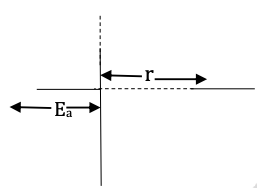E.F. on the axial line of the dipole is

$$\vec{E}_{ax}$$
= 2p / 4π𝜺0r3, r > > a

E.F. on equatorial line of the dipole is

0r3, r > > a

So,

$$\vec{E}_{ax}$$
=
$$-2\vec{E}_{eq}$$

$$\left | \vec{E}_{ax} \right | = \left ( \frac{1}{4\pi \epsilon _{0}} \right )\frac{2P}{r^{3}}$$

$$\left | \vec{E}_{eq} \right | = \left ( \frac{1}{4\pi \epsilon _{0}} \right )\frac{P}{r^{3}}$$

Question 19: Nature of equipotential surface for a point charge is –

1. a. Ellipsoid with the charge at foci.
2. b. Sphere with the charge at the centre of the sphere
3. c. Sphere with the charge on the surface of the sphere
4. d. Plane with the charge on the surface.

Solution: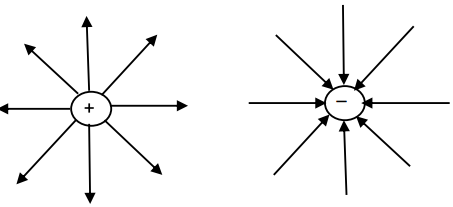For a point charge, the equipotential surface is concentric spherical shells with centre at the point charge.

Question 20: A particle of mass 1 gm and charge 1 μ C is held at rest on a frictionless horizontal surface at distance 1 m from the fixed charge 2 mC. If the particle is released, it will be repelled. The speed of the particle when it is at a distance of 10 m from the fixed charge –

1. a. 60 ms-1
2. b. 100 ms-1
3. c. 90 ms-1
4. d. 180ms-1

Solution:

Given:-

r1 = 1 m, r2 = 10m

q1 = 1 μ

q2 = 2mc

Ui = kq1q2 / r1 = [9 × 109 × 10-6 × 2 × 10-3] / 1

On solving

Ui = 18J

Ki = 0

Uf = [9 × 109 × 10-6 × 2 × 10-3] / 10 = 1.8J

Kf = 0 + (1 / 2) mv2

Kf = (1 / 2) × (0.001) × v2

Kf = 0.0005×v2

Now Apply Energy Conservation

Kf + Uf = Ki + Ui

0.0005v2 + 1.8 = 0+18

0.0005v2 = 16.2

On solving

v = 180 m/s

Question 21: A capacitor of 8F is connected as shown. Charge on the plates of the capacitor –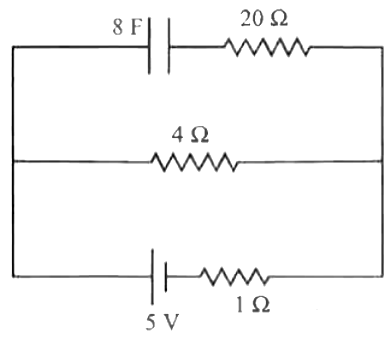1. a. 32 C
2. b. 40 C
3. c. 0 C
4. d. 80 C

Solution: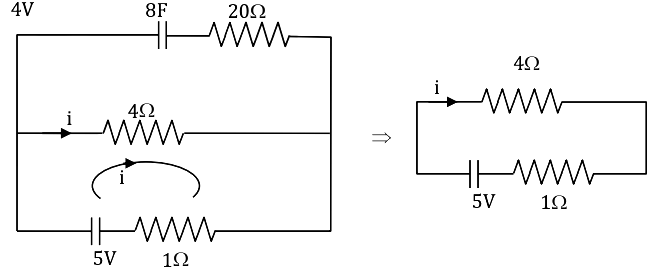No current through 20Ω

v = 4Ω * 1A

i = 5v / [4 + 1] = 1A

= 4V

VC = 4V

Q = C × V

= 8F × 4V

Q = 32C

Question 22: Four metal plates are arranged as shown. The capacitance between X and Y ( A → area of each plate, d → distance between the plates)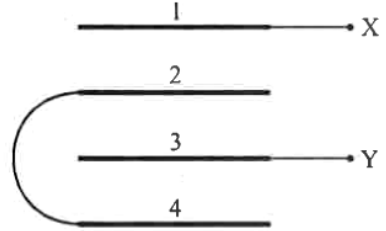1. a. (3 / 2) (𝜺0A / d)
2. b. (2𝜺0A / d)
3. c. (2 / 3) (𝜺0A / d)
4. d. (3𝜺0A / d)

Solution: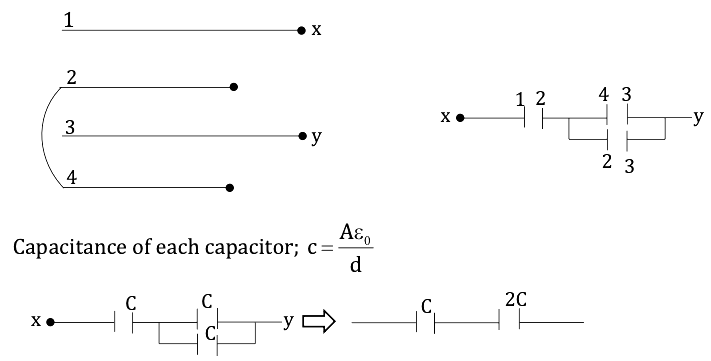Equation capacitance between x and y

Ceq = [C * 2C] / [C + 2C] = (2 / 3) C

Ceq = (2 / 3) (𝜺0A / d)

Question 23: Mobility of free electrons in a conductor is –

1. a. Directly proportional to electron density.
2. b. Directly proportional to relaxation time.
3. c. Inversely proportional to electron density.
4. d. Inversely proportional to relaxation time.

Solution:

Mobility of free – e , μ = qτ / m

μ = τ

Hence, Mobility of free e ∝ relation time.

Question 24: Variation of resistance of the conductor with temperature is as shown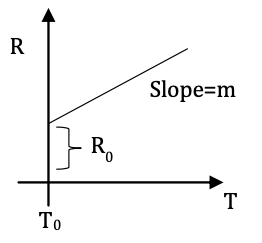The temperature coefficient (α) of the conductor is –

1. a. R0 / m
2. b. mR0
3. c. m2R0
4. d. R0 / m

Solution: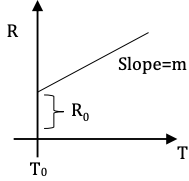Resistance of conductor, R = R0 (1 + αΔT) ---- (1)

ΔT = T - T0

From graph

R = R0 + m (T – T0)

= R0 + mΔT … (2)

Equation (1) & (2)

R0 + αΔT = mΔT

So, α = m / R0

Question 25: Potential difference between A and B in the following circuit –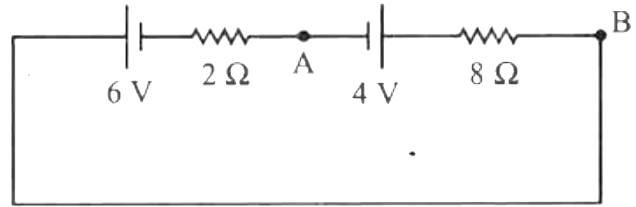1. a. 4 V
2. b. 5.6 V
3. c. 2.8 V
4. d. 6 V

Solution: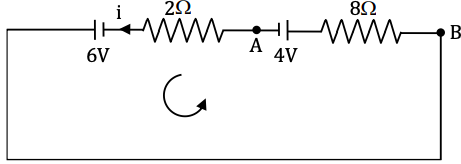Using Kirchhoff’s second law from A to B in direction of anti-clockwise.

$$I = \frac{(6-4)}{(2+8)} = 0.2A$$

$$V_{B} = (0.2\times 8) - 4 = V_{A} \Rightarrow V_{B} - V_{A} = 5.6 V$$

OR

$$V_{B} - 6 + (0.2\times 2) = V_{A} \Rightarrow V_{B} - V_{A} = 5.6 V$$

Question 26: In the following network potential at ‘O’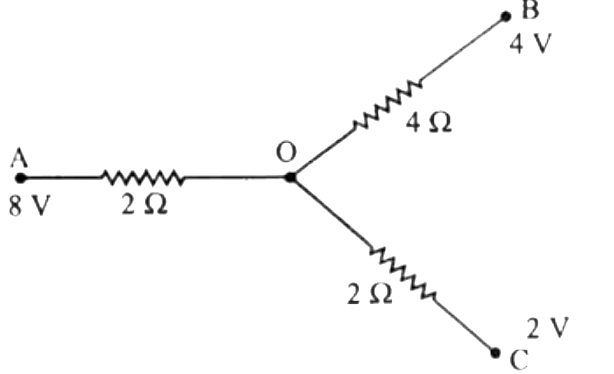1. a. 4 V
2. b. 3 V
3. c. 6 V
4. d. 4.8 V

Solution: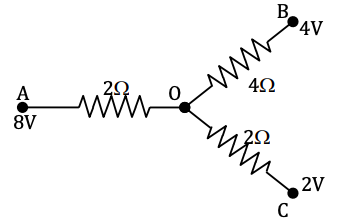Apply K.V.L.

(8 - v) / 2 = (v - 4) / 4 + (v - 2) / 2

(8 - v) / 2 = (v - 4 + 2v - 4) / 4

On solving

16 - 2v = 3v - 8

5v = 24

v = 24 / 5

v = 4.8v

Question 27: Effective resistance between A and B in the following circuit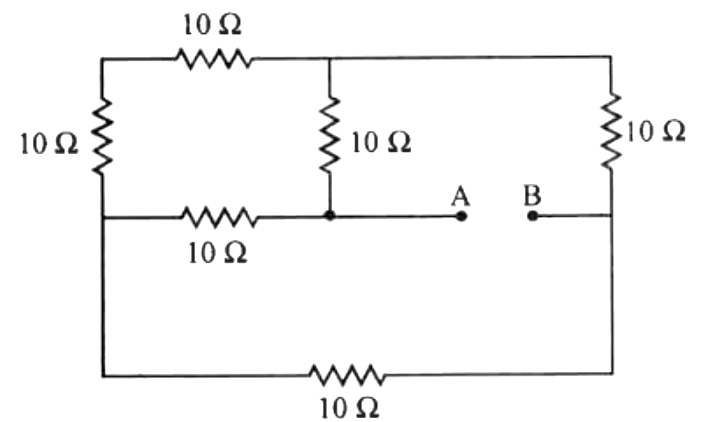1. a. 10 Ω
2. b. 20 Ω
3. c. 5 Ω
4. d. (20 / 3) Ω

Solution:The given circuit represents a well balanced wheatstone's bridge where each resistance is equal to 10 Ω.

Thus, Reg = 10 Ω

Question 28: Two heating coils of resistances 10 Ω and 20 Ω are connected in parallel and connected to a battery of emf 12V and internal resistance 1Ω. The power consumed by them are in the ratio –

1. a. 1 : 4
2. b. 1 : 3
3. c. 2 : 1
4. d. 4 : 1

Solution: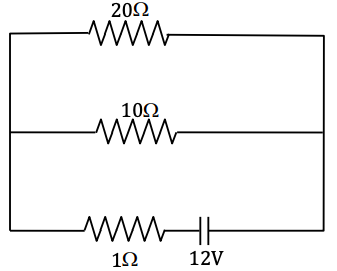Let, the potential drop across 10 Ω and 20 Ω resistor is V.

p1 = V2 / R = V2 / 10 watts

p2 = V2 / R = V2 / 20 watts

p1:p2 = 2:1

Question 29: A portion is projected with a uniform velocity ‘v’ along the axis of a current-carrying solenoid, then –

1. a. The proton will be accelerated along the axis
2. b. The proton path will be circular about the axis
3. c. The proton moves along a helical path.
4. d. The proton will continue to move with velocity ‘v’ along the axis.

Solution:

The magnetic field due to a solenoid at the axis is along the axis. Hence, if a proton is projected with a velocity ‘v’ along the axis, its velocity will be parallel to the magnetic field. If there is no component of velocity of a proton ⊥ to the magnetic field, the net force on the proton will be zero and the proton will continue to move along the axis with velocity ‘v’.

Question 30: In the cyclotron, as the radius of the circular path of the charged particle increases (ω = angular velocity, v = linear velocity)

1. a. both ω and v increases
2. b. ω only increases, v remains constant
3. c. v increases, ω remains constant
4. d. v increases, ω decreases

Solution:

v = Bqr / m

v ∝ r

Angular velocity ω = Bq / m

ω = constant

Hence, v increases, ω remains constant.

Question 31: A conducting wire carrying current is arranged as shown. The magnetic field at ‘O’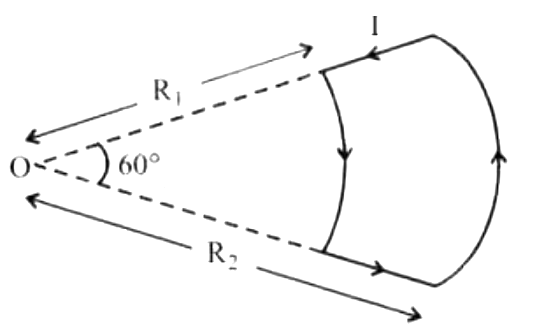1. a. [μ0i / 12] [(1 / R1) - (1 / R2)]
2. b. [μ0i / 12] [(1 / R1) + (1 / R2)]
3. c. [μ0i / 6] [(1 / R1) - (1 / R2)]
4. d. [μ0i / 6] [(1 / R1) + (1 / R2)]

Solution: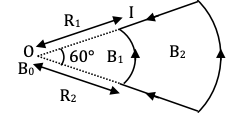Magnetic field at point ‘O’

B = [μ0 / 4π] [O1 / r] (α)

α = 60o = π / 3

B ∝ (1 / R)

B1 = [μ0I / 4πR1] [π / 3] = [μ0I / 12R1]

B2 = [μ0I / 4πR2] [π / 3] = [μ0I / 12R2]

So, magnetic field at point ‘O’ B = B1 – B2 = [μ0i / 12] [(1 / R1) - (1 / R2)]

Question 32: The quantity of a charge that will be transferred by a current flow of 20 A over 1 hour 30 minutes period is –

1. a. 10.8 × 103 C
2. b. 10.8 × 104 C
3. c. 5.4 × 103 C
4. d. 1.8 × 104 C

Solution:

Given:-

Current, I = 20A

Time, T = 1hr 30min

= 1.5 × 3600

T= 5400 sec.

q = I × t

= 20 × 5400

On solving

q = 10.8 × 104 C

Question 33: A galvanometer coil has a resistance of 50 Ω and the meter shows full-scale deflection for a current of 5 mA. This galvanometer is converted into a voltmeter of range 0 – 20 V by connecting.

1. a. 3950 Ω in series with galvanometer
2. b. 4050 Ω in series with galvanometer
3. c. 3950 Ω in parallel with galvanometer
4. d. 4050 Ω in parallel with galvanometer

Solution: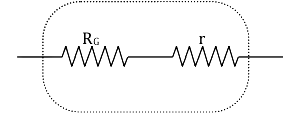Ig = 5mA = 0.005A

V = 20 volts

RG = 50Ω

V = Ig (RG + r)

20 = 0.005 (50 + r)

20 / 0.005 = 50 + r

4000 = 50 + r

R = 4000 – 50

= 3950Ω

In series with the galvanometer.

Question 34: x1 and x2 are susceptibilities of a paramagnetic material at temperatures T1K and T2K respectively, then

1. a. x1 = x2
2. b. x1T1 = x2T2
3. c. x1T2 = x2T1
4. d. x1√T1 = x2√T2

Solution:

According to curie’s law

x ∝ (1 / T)

x1 / x2 = T2 / T1

x1T1 = x2T2

Question 35: At a certain place, the horizontal component of earth’s magnetic field is 3.0 G and the angle dip at the place is 30°. The magnetic field of the earth at that location.

1. a. 4.5 G
2. b. 5.1 G
3. c. 3.5 G
4. d. 6.0 G

Solution:

Magnetic field of earth, B = BH / cos θ

Given:-

Magnetic field of horizontal component of earth

BH = 3.0G

θ = 30°

Now, value putting in equation (1)

B = 3.0 / cos 30o

B = 3.5G

Question 36: The process of superimposing message signal on the high-frequency carrier wave is called –

1. a. Amplification
2. b. Demodulation
3. c. Transmission
4. d. Modulation

Solution:

The process of superimposing a message signal on a carrier wave is modulation. High-frequency signals on the other hand can be sent over large distances with small dissipation in power.

Question 37: A long solenoid with 40 turns per cm carries a current of 1 A. The magnetic energy stored per unit volume is _____________ J/m3

1. a. 3.2π
2. b. 32π
3. c. 1.6π
4. d. 6.4π

Solution:

I = 1A

No. of turns, n = 40 turns per cm

= 4000 per m.

Now, apply the formula,

Energy stored per unit volume, (u) = μ0n2I2 / 2

u = [4π * 10-7 * 4002 * 12] / 2

On solving

u = 3.2 π J/m3

Question 38: A wheel with 10 spokes each of length ‘L’ m is rotated with a uniform angular velocity ‘ω’ in a plane normal to the magnetic field ‘B’. The emf induced between the axle and the rim of the wheel.

1. a. (1 / 2) Nω BL2
2. b. (1 / 2) ω BL2
3. c. ω BL2
4. d. Nω BL2

Solution:

Angular velocity is constant

V = 0, increase to V = ωL

Vavg = [0 + ωL] / 2

= ωL / 2

We know that

𝜺 = BVavgL

= B (ωL / 2)2

𝜺 = (1 / 2) ω BL2

Question 39: The rms value of current in a 50 Hz AC circuit is 6 A. The average value of AC current over a cycle is –

1. a. 6√2
2. b. 3 / π√2
3. c. Zero
4. d. 6 / π√2

Solution:

F = 50Hz

Irms = 6A

Iavg. = ?

Iavg = 0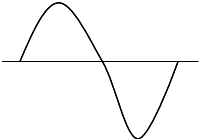As the current is negative for the same amount of time for which it is positive.

So, Average value of AC current over a cycle is zero.

Question 40: A capacitor of capacitance 10 μ F is connected to an AC source and an AC ammeter. If the source voltage varies as V = 50√2 sin 100t, the reading of the ammeter is -

1. a. 50 mA
2. b. 70.7 mA
3. c. 5.0 mA
4. d. 7.07 mA

Solution:

Given:-

C = 10 μ

= 10–5 F

V = 50√2 sin 100t

On comparing

V = V0 sin t

V0 = 50 √2

ω = 100

Now,

Vrms = 50 √2 / √2 = 50 volts

Irms = Vrms / xc

Irms = Vrms / (1 / ωc) = ωcVrms

Irms = 100 × 10–5 × 50

= 50 × 10–3 A

= 50mA

Question 41: In a series L.C.R circuit, the potential drop across L, C and R respectively are 40 V, 120 V and 60 V. Then the source voltage is –

1. a. 220 V
2. b. 160 V
3. c. 180 V
4. d. 100 V

Solution:

Given:-

VL = 40V

VC = 120

VR = 60

Source voltage, (Vs) = √(VC - VL)2 + VR2

(Vs) = √(120 - 40)2 + 602

= √802 + 602

= √6400 + 3600

= √10000

Vs = 100V

Question 42: In a series, L.C.R. circuit an alternating emf (v) and current (i) are given by the equation

v = v0 sin ωt, i0 sin (ωt + [π / 3])

The average power dissipated in the circuit over a cycle of AC is –

1. a. v0i0 / 2
2. b. v0i0 / 4
3. c. (√3 / 2) v0i0
4. d. Zero

Solution:

The power dissipated in the A.C. circuit is

P = [V0 / √2] [i0 / √2] cos φ

θ = 60o

P = [V0 / √2] [i0 / √2] cos 60o

= [V0i0 / √2] [1 / 2]

= v0i0 / 4

Question 43: Electromagnetic radiation used to sterilise milk is –

1. a. X-ray
2. b. Y-ray
3. c. UV ray

Solution:

The electromagnetic radiation used to sterilise the milk in dairy is ultraviolet.

Question 44: A plane glass plate is placed over various coloured letters (violet, green, yellow, red). The letter which appears to raise more.

1. a. Red
2. b. Yellow
3. c. Green
4. d. Violet

Solution:

Normal shift through the plate of thickness t

d = t (1 - [1 / μ])

Now, Refractive index (μ) is related to wavelength (λ) of light as

μ = A + (B / λ2)

Question 45: A ray of light passes through four transparent media with refractive index n1, n2, n3 and n4 as shown. The surfaces of all media are parallel.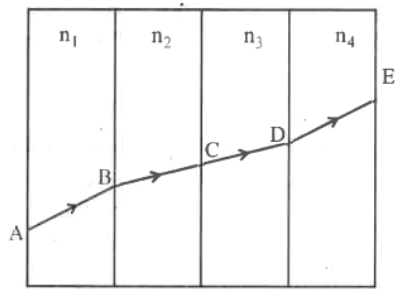If the emergent ray DE is parallel to incident ray AB, then

1. a. n1 = n4
2. b. n2 = n4
3. c. n3 = n4
4. d. n1 = [n2 + n3 + n4] / 3

Solution: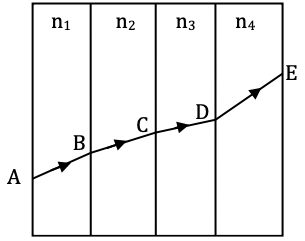Angle of incidence = Angle of refraction

i = r … (1)

Using snell’s law of refraction

n1sini = n4sini

n1 = n4

Question 46: Focal length of a convex lens is 20 cm and its RI is 1.5. It produces an erect, enlarged image if the distance of the object from the lens is –

1. a. 40 cm
2. b. 30 cm
3. c. 15 cm
4. d. 20 cm

Solution:

A convex lens forms an erect and enlarged image when the object is placed between focus and the lens. So, object distance must be less than the focal length of the lens. Thus, object distance must be 15cm.

Question 47: A ray of light suffers a minimum deviation when incident on an equilateral prism of refractive index √2. The angle of incidence is –

1. a. 30°
2. b. 45°
3. c. 60°
4. d. 50°

Solution: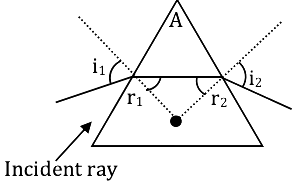n1 = 1, n2 = √2

A = 60° (equilateral prism)

By geometry A = r1 + r2 … (1)

For min. deviation A = r1 = r2 … (2)

From equation (1) & (2)

A = 2r1

R1 = 60 / 2 = 30°

Using snell’s law

n1 = sin i1 = n2 sin (r1)

1.sin i1 = √2 (1 / 2)

After solving

i1 = 45°

Question 48: In Young’s double-slit experiment the source is white light. One slit is covered with a red filter and the other with a blue filter. There shall be –

1. a. Alternate red & blue fringes
2. b. Alternate dark & pink fringes
3. c. Alternate dark & yellow fringes
4. d. No interference

Solution:

The light from two slits of young’s double-slit experiment is of different colours, and having different wavelengths and frequencies.

Hence, there shall be no interference fringes.

Question 49: Light of wavelength 600 𝜂 m is incident normally on a slit of width 0.2 mm. The angular width of central maxima in the diffraction pattern is (measured from minimum to maximum).

1. a. 6 × 10-3 rad
2. b. 4 × 10-3 rad
3. c. 2.4 × 10-3 rad
4. d. 4.5 × 10-3 rad

Solution:

λ = 600 nm

= 600 × 10–9m

Linear width of central maxima is = 2λD / a

Angular width = 2λ / d

d = 0.2mm = 0.2 × 10–3m

So,

Angular width = 2 [600 × 10–9] / [0.2 × 10–3]

After solving Angular width = 6 × 10-3 rad

Question 50: For what distance is ray optics is a good approximation when the aperture is 4 mm and the wavelength of light is 400 𝜂 m?

1. a. 24 m
2. b. 40 m
3. c. 18 m
4. d. 30 m

Solution:

Aperture width a = 4mm

= 4 × 10-3m

Wavelength

λ = 400nm

= 400 × 10-9 m

So, Fresnel distance:-

Df = a2 / λ

= (4 * 10-3)2 / (400 * 10-9)

After solving

Df = 40m

Question 51: The variation of photocurrent with collector potential for different frequencies of incident radiation v1, v2 and v3 is as shown in the graph, then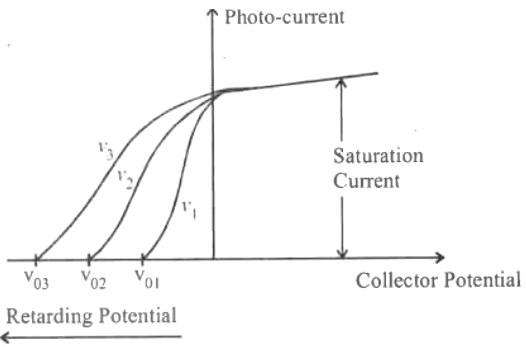1. a. v1 = v2 = v3
2. b. v1 > v2 > v3
3. c. v1 < v2 < v3
4. d. v3 = (v1 + v2) / 2

Solution: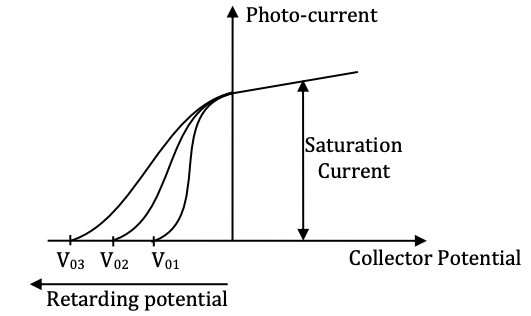V03 > V02 > V01 [stopping potential]

Minimum Energy of photon required for emission ‘α’ stopping potential

E3 > E2 > E1

E = h

So,

V3 > V2 > V1

Question 52: The de Broglie wavelength of an electron accelerated to a potential of 400 V is approximately –

1. a. 0.03 nm
2. b. 0.04 nm
3. c. 0.12 nm
4. d. 0.06 nm

Solution:

Using de-Broglie wavelength

λ = (123 / √v) Å

Given:-

V = 400v

λ = [12.3 / √400] = (12.3 / 20) Å

= 0.615A°

λ = 0.0615 nm

Question 53: Total energy of an electron in an excited state of a hydrogen atom is – 3.4 eV. The kinetic and potential energy of an electron in this state –

1. a. K = -3.4 eV U = - 6.8 eV
2. b. K = 3.4 eV U = - 6.8 eV
3. c. K = -6.8eV U = 3.4eV
4. d. K = 10.2eV U = - 13.6eV

Solution:

–3.4ev = K.E + P.E. ….. (i) [Given]

The relation b/w P.E. & K.E

P.E. = –2 K.E. ......... (ii)

Use the relation in equation (i)

–3.4ev = K.E. – 2K.E.

K.E. = 3.4ev

From equation (ii)

P.E. = –2 (3.4ev)

P.E. = –6.8ev

Question 54: When an electron jumps from n = 4 level to n = 1 level, the angular momentum of electron changes by –

1. a. h / 2π
2. b. 2h / 2π
3. c. 3h / 2π
4. d. 4h / 2π

Solution:

From Bohr’s postulate, the angular momentum is:-

mvr = nh / 2π

For n = 4

mvr = 4h / 2π …(i)

For n = 1

mvr = h / 2π …(ii)

Thus, the change in angular momentum

Δmvr = [4h / 2π] - [h / 2π]

Δmvr = 3h / 2π

Question 55: A radioactive sample of half-life 10 days contains 1000 x nuclei. Number of original nuclei present after 5 days is –

1. a. 707 x
2. b. 750 x
3. c. 500 x
4. d. 250 x

Solution:

Nuclei present after 5 days, n = No exp [-0.693 / (T2 / 2)]t … (1)

Given:-

No = 1000x

T1/2 = 10days

t = 5days

Substitute’s values in equation (1)

N = 1000x × [-0.693 * 5 / (10)]

N =1000x × exp. (–0.346)

N = 1000x × 0.707

N = 707x

Question 56: An element X decays into element Z by a two-step process.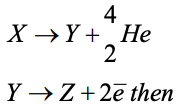1. a. X & Z are isobars.
2. b. X & Y are isotopes.
3. c. X & Z are isotones.
4. d. X & Z are isotopes.

Solution:

A & Z = mass number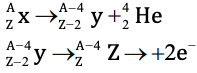x & z are isotopes.

Question 57: A nucleus of mass 20 u emits a γ photon of energy 6 MeV. If the emission assume to occur when the nucleus is free and rest, then the nucleus will have kinetic energy nearest to (Take 1u = 1.6 × 10-27 kg)

1. a. 10 KeV
2. b. 1 KeV
3. c. 0.1 KeV
4. d. 100 KeV

Solution:

E = 6Mev

= 6 × 1.6 × 10-13J

m = 20u

= 20 × 1.6 × 10-27 kg

Momentum of γ photon,

P = E / C

P = E / C [momentum of Nucleus]

K = P2 / 2m = E2 / 2mc2

K = [6 × 1.6 × 10-13]2 / [2 × (20 × 1.6 × 10-27 ) × (3 × 108)2]

After solving

K.E. = 1Kev

Question 58: Constant DC voltage is required from a variable AC voltage. Which of the following is the correct order of operation?

1. a. Regulator, filter, rectifier
2. b. Rectifier, regulator, filter
3. c. Rectifier, filter, regulator
4. d. Filter, regulator, rectifier

Solution:

To convert AC to DC, a full-wave rectifier is required; the O/P of the full-wave rectifier will be positive cycles of the applied input AC voltage. To convert the positive cycles to ripples a RC filter will be required.

Question 59: In a transistor, the collector current varies by 0.49 mA and emitter current varies by 0.50 mA. Current gain β measured is –

1. a. 49
2. b. 150
3. c. 99
4. d. 100

Solution:

Current gain β = ΔIc / ΔIb ---- (1)

Given

ΔIc = 0.49mA

ΔIe = 0.50mA [emitter current]

Change in base current:-

ΔIb = ΔIe – ΔIc

= 0.50 –0.49

= 0.01mA

Substitute value in equation (1)

β = 0.49 / 0.01 = 49

Question 60: Identify the logic operation carried out by the following circuit.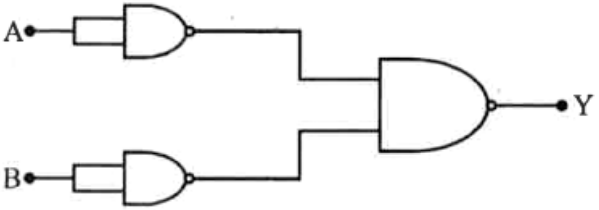1. a. AND
2. b. NAND
3. c. NOR
4. d. OR

Solution: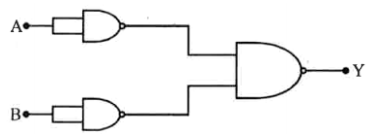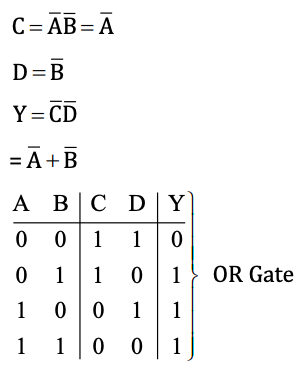### KCET 2016 Physics Question Paper with Solutions